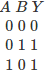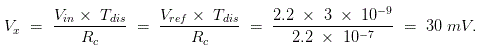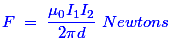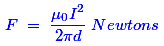# MCQs on Digital Electronics

##### Page 9 of 35. Go to page 1 2 3 4 5 6 7 8 9 10 11 12 13 14 15 16 17 18 19 20 21 22 23 24 25 26 27 28 29 30 31 32 33 34 35
01․ What will be the Boolean expression for an inverter logic gate, where input is A and output is G ?
G = A
A= G
G = Ā
None of the above

Because output is the inversion of the input A. ∴ G = Ā.

02․ When will be the output of an exclusive-OR gate is HIGH ?
The inputs are different
All inputs are LOW
All inputs are HIGH
The inputs are equal

This means that the output from this gate will be a 1 only when one or the other of the inputs is 1.03․ Dual slope ADC has R = 1 KΩ and C = 0.22 nanofarad has charging and discharge times for some voltage is 9 ns and 3 ns respectively. The reference Voltage is 2.2 V. What is the peak voltage reached by triangular wave during charging?
90 mV.
30 mV.
300 mV.
900 mV.04․ Which of the following logic circuits is the fastest ?
RTL.
DTL.
TTL.
All have same speed.

Since, the transistors of a standard TTL gate are saturated switches. The minority carrier storage time in each junction limits the switching speed of the device. Variations on the basic TTL design are intended to reduce these effects and improves speed or lower power consumption. Its switching speed can be arround 100 MHz.

05․ Which of the following is not a transmission medium?
Telephone lines.
Coaxial cables.
Modem.
Microwave systems.

Modem means modulator and demodulator. This is used to get the internet connection from the hub. Hence, it does not help to transmit or receive the messages as a transmission medium.

06․ The two conductors of a transmission line carry equal current I in opposite directions. The force on each conductor is
proportional to I.
proportional to I2.
proportional to distance between the conductors.
inversely proportional to I2.

We know that the force is basically given by the following expression.If the two conductor currents I1 and I2 are equal and if they are in opposite direction, then the force developed by the conductors can be written as,Hence, according to the above expression of force, the force is directly proportional to the square of the currents and the force is repulsive in nature.magnetic field

07․ A single flip-flop can be cleared (reset) to
1
0
Both A and B
None

Reset state means Q = 0 and set state means Q' = 1

08․ The time required for a gate to change its output is called as
Decay time
start time
run time
propagation time

The time required to change the output of a gate is known as the propagation delay.

09․ Choose the option which is not a code conversion standard
BCD code
Excess-3 code
Gray code
Truth code

There is no such code for conversion by the name "truth code". Rest of the codes binary coded decimal, excess 3 code and gray codes are used for conversion of binary numbers.

10․ At which the digital data can be applied to a a gate is known as ________ frequency.
Propagation
Truth
Operating
Run-time

Operating frequency is the rating at which the gate will perform its function with full efficiency.

<<<7891011>>>